V.6 No 1 51 On the wave-particle duality4. Interaction of a chain of electrons with dynamic field of atom Basing on the drawn pattern of dynamic field of atom, we can study the interaction of the electron beam with this field. Suppose, a chain of low-energy electrons hits the region of the atom field at the distance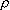from the centre, as is shown in Fig. 15.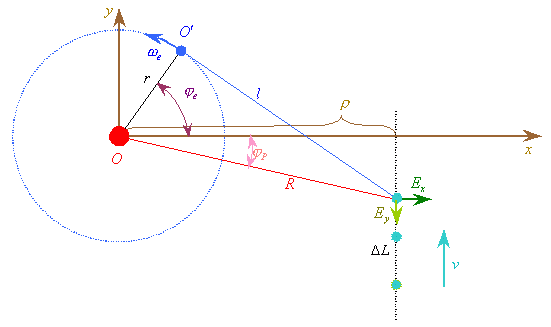Fig. 15. Interaction of electron chain with dynamic field of atom
 Noting the expressions (39) having been found before for dynamic field of an atom, the modelling equations describing the interaction of each electron with this dynamic field, in the premise of thermal speed of electrons, can be written in the following way: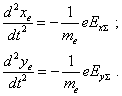(40)
 Noting also the little radius of field and premising the electron beam to be monochromatic, we can solve numerically the system of equations (40) jointly with (39). The result of this calculation is shown in the dynamic diagram, Fig. 16.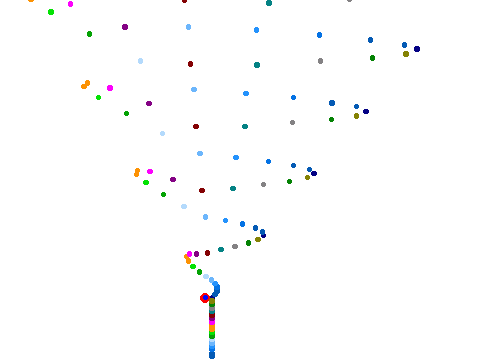Fig. 16. Dynamic diagram of electron chain interaction with the dynamic field of atom;= 50 r , r = 2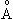, v = 4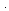10 4 m / s
 The diagram shows that from the interaction of electrons with dynamic field of atom, the periodic wave-like structure propagating in space behind the atom is formed. This structure has a period equal to that of electron’s orbiting and the wavelength proportional to the product of electrons’ speed by the orbital period of electron. At the same time, the speeds of electrons after interaction do not remain unchanged; this predestines the gradual distortion of the wave pattern. It is also typical that each electron scatters in accordance with the Rutherford model, which interrelates this scattering with Rutherford’s model. The difference is, the field phase affecting each electron is different in this model; this causes the formation of wave-like structure in space and time. As we see from the diagram, as the result there forms some discrete periodic structure without smooth transitions between the regions of extremes of density of electrons, though this situation will considerably change in case of non-monochromatic electron beam and variation of the distance to the target. In case of interaction of some assemblage of such chains with the periodical (or quasi-periodical) structure, the superimposition of wave-like patterns forms the redistribution of electron density in space similar to the interference patterns for EM waves. If we furthermore premise a considerable lessening of the density of electrons in the chain and variation of the distance to the target and of speed of electrons, the probability that electron will hit to a definite part of screen will be proportional to the period of electron’s orbiting with account of the above factors, which will be equivalent to the resonance interaction without any doubtful wave functions of quantum-mechanical formalism.

Contents: / 43 / 44 / 45 / 46 / 47 / 48 / 49 / 50 / 51 / 52 /